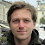## Wednesday, May 9, 2012

### Existing occurrences of lattices

As already pointed out by Dmitrii Pasechnik in the comments to the project proposal, one of the first things to do is probably to search for existing occurrences of lattices in Sage.

A bold search for "lattice" in *.py and *.pyx files in sage-5.0b11/sage/sage yields 3,946 matches. Here's what they are about (places with potential overlap with or use for our new Lattice class in bold):
• algebras.quatalg.basis_for_quaternion_lattice: returns a basis for the `\\ZZ`-lattice in a quaternion algebra spanned the given generators. It uses _hnf_pari (which calculates the upper triangular Hermite normal form) of an integer matrix.
• categories.*: "lattice" in the sense of a partially ordered set with unique supremum.
• coding.code_constructions.ToricCode
• combinat.*: poset.
• crypto.lattice.gen_lattice: generates different types of lattice bases of row vectors relevant in cryptography.
• geometry: lattice polytopes, integral points. Using the PALP library.
• group.abelian_gps.abelian_group.subgroup_reduced: find small basis.
• homology.simplicial_complex.lattice_paths:
• interfaces.r: poset.
• libs.fplll: wrapper for the floating-point LLL implementation in fplll. This library also contains a floating-point implementation of the Kannan-Fincke-Pohst algorithm that finds a shortest non-zero lattice vector, and the Block Korkin-Zolotarev (BKZ) reduction algorithm.
• libs.ntl.ntl_mat_ZZ.LLL (and variants): LLL implementation with different precisions (up to arbitrary precision floats).
• libs.pari.gen: period lattices of elliptic curves.
• matrix.matrix_integer_dense: LLL and BKZ functions (using fplll or ntl).
• modular.abvar.*: calculate lattices that define various subgroups etc.
• modular.modsym.ambient.ModularSymbolsAmbient.integral_structure
• modular.quatalg.brandt: orders and ideals in quaternion algebras.
• modular.etaproducts.EtaGroup_class.basis: uses LLL algorithm.
• modules.fg_pid.fgp_module: lattice used in example code.
• modules.free_module.FreeModule_generic_pid.index_in: lattice_index.
• rings.number_field.CyclotomicField._positive_integral_elements_with_trace: enumerate lattice points.
• rings.number_field.order: lattice index.
• rings.number_field.totallyreal_data.hermite_constant: nth Hermite constant.
• rings.number_field.totallyreal_rel.integral_elements_in_box: uses geometry.lattice_polytope.LatticePolytope to find points numerically.
• rings.number_field.enumerate_totallyreal_fields_prim: find a minimal lattice element.
• rings.polynomial_modn_dens.small_roots: uses LLL algorithm.
• rings.qqbar: poset (lattice of algebraic extensions).
• rings.rational_field: example code using period lattice of elliptic curves.
• schemes.elliptic_curves: period lattice of elliptic curves.
• schemes.generic.*: lattice polytopes.
Do you know of any other relevant places where lattices are used in Sage? Any ideas on how any of these pieces of code might benefit from a new Lattice class?

1.Hi, Jan:

Here a PhD student who has used Sage for 6D crystallographic groups.

Also in categories.* I think you have Cartan types and Crystals, both of which define lattices (though some of the Cartan types define the same one, like G_2 and A_2; I have a list of those coincidences for dimension/rank up to 3).

Also, at some point it would be nice to have lattices as a subobject of Bravais types, which are (not exactly) a vector space of matrices. But I guess that's too much for a summer.

For whatever you may need, my mail is jesus.torrado at the server tum.de.

Servus!

2.